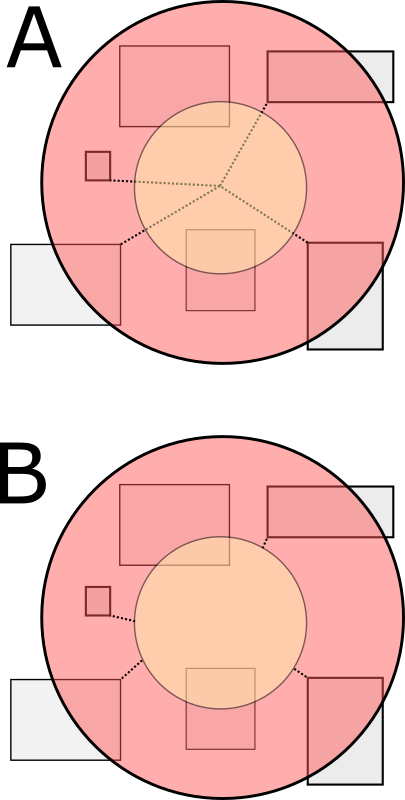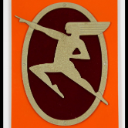# Distance calculation

159
1
05-02-2019 01:56 AMOccasional Contributor

Hi all

I am struggling with how to return a distance from a drawn geometry (polygon, extent, circle) that is buffered (user specified distance) and which then selects any features within that object. At the moment, I get the distance from the centre point of the object (A) and not the edge of the buffer start edge (B). Hopefully the following graphic should illustrate better what I need to calculate:Here is the code I am using (Version 3.28 api):

/* function to create toolbar drawing shapes */
function initToolbar(evt) {
tb = new Draw(map);

// event delegation so a click handler is not needed for each individual button
on(dom.byId("info"), "click", function(evt) {
if ( evt.target.id === "info" ) {
return;
}

var tool = evt.target.id.toLowerCase();
tb.activate(tool);
});
}

//deactivate the toolbar and clear existing graphics
tb.deactivate();
var geometry = evt.geometry;

var params = new BufferParameters();
params.distances = [dom.byId("distance").value];
params.outSpatialReference = map.spatialReference;
params.unit = GeometryService[dom.byId("unit").value];

var symbol;

if ( geometry.type === "point" || geometry.type === "multipoint") {
userMP = evt.geometry;
symbol = markerSymbol;
} else if ( geometry.type === "line" || geometry.type === "polyline") {
userMP = geometry;
symbol = lineSymbol;
} else if ( geometry.type === "extent") {
userMP = geometry.getCenter();
symbol = lineSymbol;
}
else {
userMP = geometry.getCentroid();
symbol = fillSymbol;
}

params.geometries = [geometry];

esriConfig.defaults.geometryService.buffer(params, showBuffer);

function showBuffer(bufferedGeometries) {
var symbol = new SimpleFillSymbol(
SimpleFillSymbol.STYLE_SOLID,
new SimpleLineSymbol(
SimpleLineSymbol.STYLE_SOLID,
new Color([255,0,0,0.65]), 2
),
new Color([255,0,0,0.35])
);

arrayUtils.forEach(bufferedGeometries, function(geometry) {
var graphic = new Graphic(geometry, symbol);
});

}

on(esriConfig.defaults.geometryService, "buffer-complete", function(Geom){
queryMapService(Geom)
})

/* Function to query layers based on tool geometry chosen e.g. polygon, circle etc */
function queryMapService(Geom){
var promises = [];

/* query for feature layers */
var query = new Query();
query.outFields = ["*"];
query.returnGeometry = false;
query.geometry = Geom.geometries;

function findClosestIBAs(features) {
var geometryService = new GeometryService("../arcgis/rest/services/Utilities/Geometry/GeometryServer");

var promises;
var dlist = [];
var list = [];
var rstr = "";

var ptloc, distp;

for (var x = 0; x < features.length; x++) {
var distParams = new DistanceParameters();
distParams.distanceUnit = GeometryService.UNIT_KILOMETER;
distParams.geometry1 = userMP;
distParams.geodesic = true;
SitRecID = features.attributes["SitRecID"];
IntName = features.attributes["IntName"];
ptloc = features.geometry;
distParams.geometry2 = features.geometry;
dlist.push({name:IntName + " " + SitRecID, loc:ptloc});

distp = geometryService.distance(distParams);
list.push(distp)
}
All(list).then(lang.hitch(this,function(results){
var shortestDist = Number.POSITIVE_INFINITY;
var closestBlock = "";
for (var dv = 0; dv < dlist.length; dv++){
appendSensIBAs(dlist[dv].name + " is " + results[dv].toFixed(2) + "km");
rstr += dlist[dv].name + " is " + results[dv].toFixed(2) + "km";
if(results[dv] < shortestDist){
shortestDist = results[dv];
closestBlock = dlist[dv].name;
}
}

}));
}

Any ideas or suggestions gratefully received!by``distParams.geometry1 = userMP; ‍``